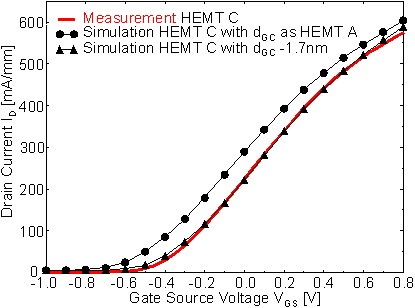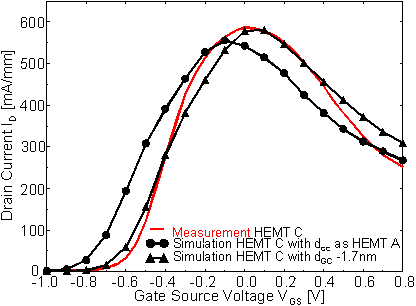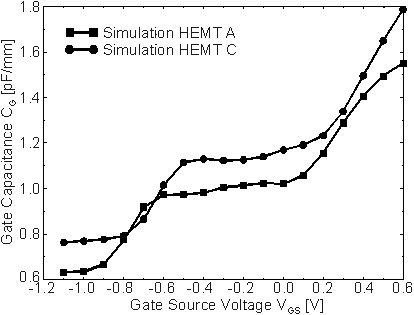Next: 6.1.2 RF characteristics Up: 6.1.1 DC Characteristics Previous: 6.1.1.1 Comparison of HEMT A and HEMT B

6.1.1.2 Comparison of HEMT A and HEMT C

Initially, HEMT C was assumed to possess the same distance dGC = 25 nm as HEMT A but only a slightly longer LG = 190 nm and a shorter LR = 60 nm. When these values are used in the simulation, this results in a too negative value of VT and an overestimated ID, as can be seen in  Figure 6.10. However, a reduction of dGC by 1.7 nm leads to perfect coincidence of measured and simulated curves, as it is also shown in  Figure 6.10.Figure 6.10 Measured (bold line without symbols) and simulated transfer characteristics of HEMT C with the nominal dGC (circles) and dGC - 1.7 nm (triangles) at VDS = 2.0 V

Variations of dGC of such small size can easily occur in different technology runs. The agreement between measurement and simulation is even more evident for the transconductance shown in  Figure 6.11. Both the peak value gm max = 580 mS/mm and its occurrence at VGS = 0 V are simulated very well.Figure 6.11 Measured (bold line without symbols) and simulated transconductance of HEMT C with the nominal dGC (circles) and dGC - 1.7 nm (triangles) at VDS = 2.0 V

We now return to the initial simulation of HEMT C which was performed under the assumption that dGC is equal to the value of HEMT A (dgc = 25 nm). When this simulation of a "hypothetical" HEMT C (circles in  Figure 6.11) is compared to the simulation of HEMT A in  Figure 6.7, it is found that the hypothetical device has a gm max that is about 25 mS/mm larger than the value of HEMT A though its gate is 20 nm longer. This must be a consequence of LR which is 75 nm shorter and obviously overcompensates the small effect of the slightly longer gate.

From the previous comparison between HEMTs A and B (which share the same LR), we can estimate that an LG increase of 20 nm only causes a negligible decrease of gm max of about 5 mS/mm. This independently confirms the conclusion drawn above: the reduction of LR had a stronger impact on the transconductance of HEMT C than the slightly longer LG.

A drawback of the shrinkage of LR is the increase of CG. This can be seen in  Figure 6.12 which shows the simulated gate capacitance CG of HEMTs A and C. For HEMT C, the whole function CG(VGS) is shifted towards higher values by about 130 fF/mm as compared to HEMT A. As the shift is already completely present in the pinchoff region it can be entirely attributed to the increased coupling between the gate and drain contacts.Figure 6.12 Simulated CG of HEMT A (squares) and HEMT C (circles) at VDS = 2.0 VNext: 6.1.2 RF characteristics Up: 6.1.1 DC Characteristics Previous: 6.1.1.1 Comparison of HEMT A and HEMT B

Helmut Brech
1998-03-11